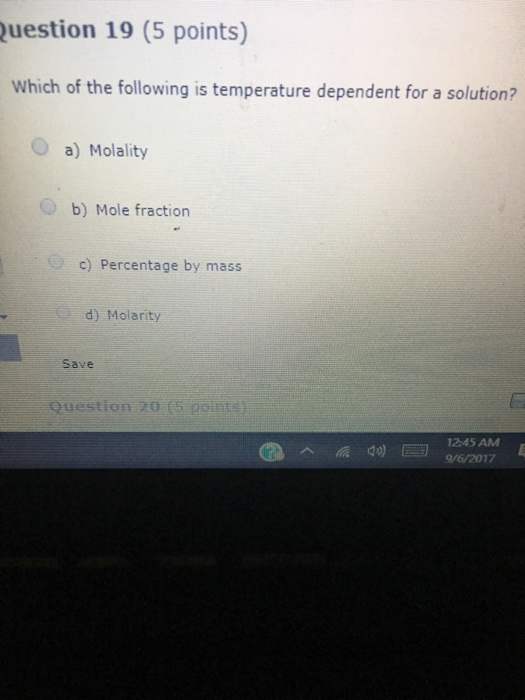# Homework Solution: Which of the following is temperature dependent for a solution? a) Molality b)…Which of the following is temperature dependent for a solution? a) Molality b) Mole fraction c) percentage by mass d) Molarity